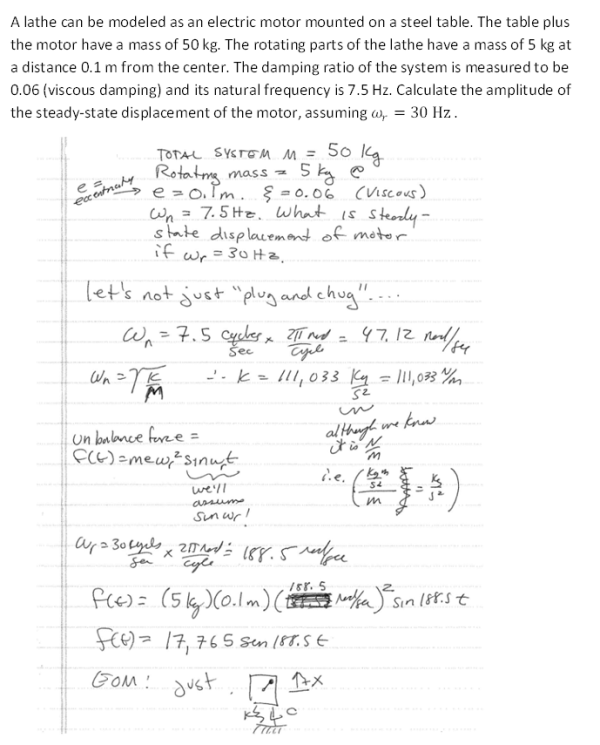A lathe can be modeled as an electric motor mounted on a steel table. The table plus the motor have a mass of 50 kg. The rotating parts of the lathe have a mass of 5 kg at a distance 0.1 m from the center. The damping ratio of the system is measured to be 0.06 (viscous damping) and its natural frequency in 7.5 Hz. Calculate the amplitude of the steady-state displacement of the motor, assuming wr=30 Hz.A lathe can be modeled as an electric motor mounted on a steel table. The table plus the motor have a mass of 50 kg. The rotating parts of the lathe have a mass of 5 kg at a distance 0.1 m from the center. The damping ratio of the system is measured to be 0.06 (viscous damping) and its natural frequency in 7.5 Hz. Calculate the amplitude of the steady-state displacement of the motor, assuming wr=30 Hz.

Vibrations Page 1 vibrations vibrations vibrations vibrations vibrations vibrations vibrations Vibrations Page 2